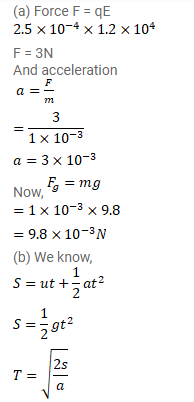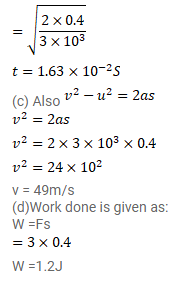# A particle of mass 1 g and charge

Question:

A particle of mass $1 \mathrm{~g}$ and charge $2.5^{\times 10^{-4}} \mathrm{C}$ is released from rest in an electric field of $1.2^{\times 10^{-4}} \mathrm{~N} / \mathrm{C}$.

(a) Find the electric force and the force of gravity acting on this particle. Can one of these forces be neglected in comparison with the other for approximate analysis?

(b) How long will it take for the particle to travel a distance of $40 \mathrm{~cm}$ ?

(c) What will be the speed of the particle after travelling this distance?

(d) How much is the work done by the electric force on the particle during this period?

Solution: